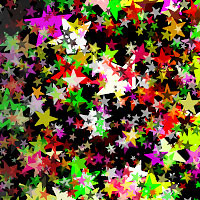Implicit and Explicit Procedural Noise
By Neil Blevins
Apr 3rd 2015

A quick little discussion on Implicit and Explicit Procedural Noise. I've been playing around a bit using Allegorithmic's Substance Designer, and they use "Explicit Noise". I know the difference between Implicit and Explicit modeling and shading, but had never heard of "Explicit Noise" before. Upon finding almost no literature on the web on the subject, I did some digging and here's the result.

First, a very basic definition of Implicit and Explicit...

1) An Implicit thing in CG is where a mathematical equation can represent something.
2) An Explicit thing in CG is where you have a good approximation of that something.

So for example, in geometry, an implicit model is where it calculates the sphere as an equation, a standard sphere equation would be x^2 + y^2 + z^2 = 1. So if you want to get the xyz point anywhere on that sphere, it can be calculated to an exact position.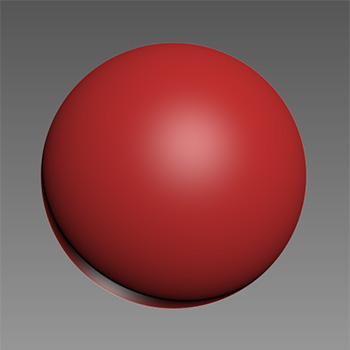A polygonal sphere is an explicit model, because you only have an approximation of a true sphere. Even if you increase the number of polygons, you're just getting a better and better approximation, you will never actually reach a perfect sphere at any point.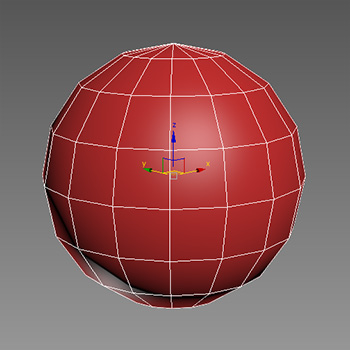In texturing, a standard procedural noise is implicit (for example, Perlin noise), because when you zoom in, it just calculates more of the image based on the original algorithm, so you never reach a point where you can't zoom in any more.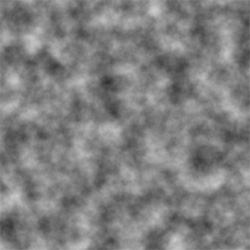Something like a bitmap is an explicit image, since the result is stored as a set of pixels, if you zoom in past the pixel resolution of the image, you don't get more information, and it just looks all pixelated. Its an approximation of the original implicit noise.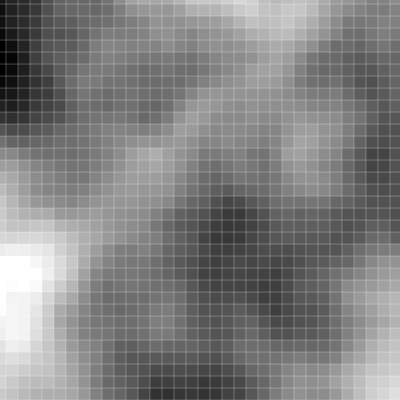So what is "Explicit Noise" in Substance Designer? While they don't give the exact formula, basically their noises are made up by taking an image and repeatedly placing it on a 2d surface, each randomly rotated and placed, then the image is subdivided and the process repeats. For more info, read here: http://support.allegorithmic.com/documentation/display/SD5/FXMaps?src=contextnavpagetreemode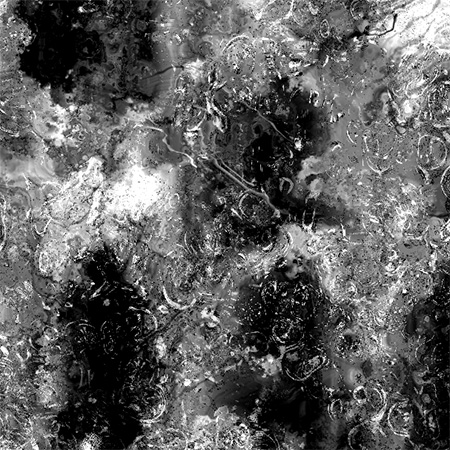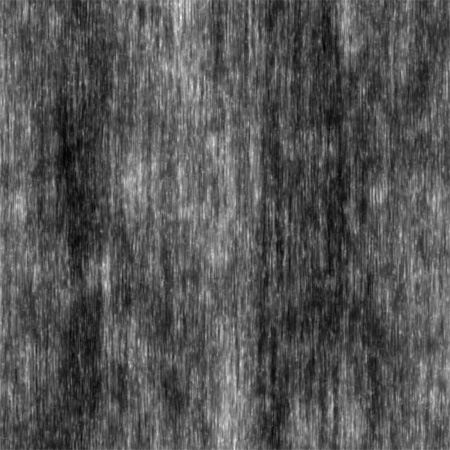This can create patterns that are very similar to implicit procedural noise, but with a few different advantages and disadvantages.

• One advantage is you can create a larger number of noise looks because you're starting with any arbitrary image.
• The noises can also be seamless.